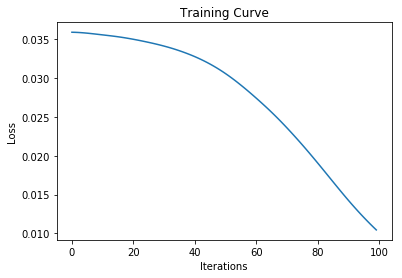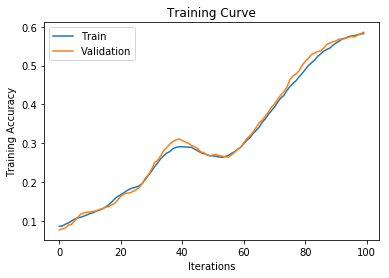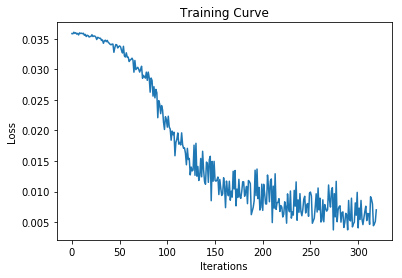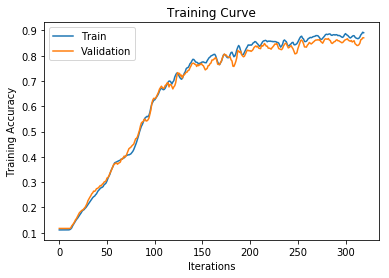# Classification in PyTorch¶

In this section, we're going to look at actually how to define and debug a neural network in PyTorch. We will also take the opportunity to go beyond a binary classification problem, and instead work on a more general classification problem

Let's start, as always, with our neural network model from last time.

In :
import torch
import torch.nn as nn
import torch.nn.functional as F

class Model(nn.Module):
def __init__(self, num_hidden):
super(Model, self).__init__()
self.layer1 = nn.Linear(28 * 28, num_hidden)
self.layer2 = nn.Linear(num_hidden, 1)
self.num_hidden = num_hidden
def forward(self, img):
flattened = img.view(-1, 28 * 28)
activation1 = self.layer1(flattened)
activation1 = F.relu(activation1)
activation2 = self.layer2(activation1)
return activation2

model = Model(30)


The module torch.nn contains different classess that help you build neural network models. All models in PyTorch inherit from the subclass nn.Module, which has useful methods like parameters(), __call__() and others.

This module torch.nn also has various layers that you can use to build your neural network. For example, we used nn.Linear in our code above, which constructs a fully connected layer. In particular, we defined two nn.Linear layers as part of our network in the __init__ method. Next week, we'll start to see other types of layers like nn.Conv2d.

(What exactly is a "layer"? It is essentially a step in the neural network computation. We can also think of the ReLU activation as a "layer". However, there are no tunable parameters associated with the ReLU activation function. We don't need to keep track of "states" associated with the ReLU acitvation, so it is not initalized as a "layer" in the __init__ function.)

The __init__ method is where we typically define the attributes of a class. In our case, all the "sub-components" of our model should be defined here, along with any other setting that we wish to save -- for example self.num_hidden.

The forward method is called when we use the neural network to make a prediction. Another term for "making a prediction" is running the forward pass, because information flows forward from the input through the hidden layers to the output. When we compute parameter updates, we run the backward pass by calling the function loss.backward(). During the backward pass, information about parameter changes flows backwards, from the output through the hidden layers to the input.

The forward method is called from the __call__ function of nn.Module, so that when we run model(input), the forward method is called.

In our case, the forward function does the following:

1. "Flatten" the input parameter img. The parameter img is a PyTorch tensor of dimension batch_size x 28 x 28, or [-1, 28, 28] (or possibly [-1, 1, 28, 28]). The dimension size -1 is a placeholder for a "unknown" dimension size. After flattening, the variable flattened will be a PyTorch tensor of dimension [-1, 28*28].
2. Run the forward pass of self.layer1, which computes activations of our hidden layer given our flattened input.
3. Pass those activations (activation1) through the ReLU nonlinearity.
4. Run the forward pass of self.layer2, which computes activations of our output layer given activation2.

Note that in the last few classes, we have used the sigmoid activation function to turn the final activation2 value into a probability. This step is not a part of the forward method. The reason is that the computation of the loss function is more numerically stable when we don't run the sigmoid function (we get a more accurate loss function value because of the way floating-point values are represented on the computer).

## Define a Neural Network¶

To define our own neural network, we should understand the inputs and outputs that are expected. For a binary-classification problem, our output can be a single neuron. We should then decide on the architecture(s) that we want. How many layers should we have? How many neurons in each layer? And later on -- what kind of layers will we use?

Here is an example of a 4-layer neural network that performs binary classification on a 28x28 image.

In :
class Model(nn.Module):
def __init__(self, num_hidden):
super(Model, self).__init__()
self.layer1 = nn.Linear(28 * 28, 100)
self.layer2 = nn.Linear(100, 50)
self.layer3 = nn.Linear(50, 20)
self.layer4 = nn.Linear(20, 1)
self.num_hidden = num_hidden
def forward(self, img):
flattened = img.view(-1, 28 * 28)
activation1 = F.relu(self.layer1(flattened))
activation2 = F.relu(self.layer2(activation1))
activation3 = F.relu(self.layer3(activation2))
output = self.layer4(activation3)
return output


Notice that in a fully-connected feed-forward network, the number of units in each layer always decreases. The neural network is forced to condense information, step-by-step, until it computes the target output we desire. When solving prediction problems, we will rarely (if ever) have a later layer have more neurons than a previous layer.

## N-ary Classification¶

For the rest of this chapter, let's work on a slightly different classification problem. Instead of a binary classification problem, we will work on a general classification problem, where an input value will be classified into one of many categories.

We will perform the digit classification problem: given an image of a hand-written digit, we will predict what digit the image represents. We are already familiar with the MNIST data, but here it is again:

In :
import matplotlib.pyplot as plt
from torchvision import datasets, transforms

for k, (image, label) in enumerate(mnist_images):
if k >= 18:
break
plt.subplot(3, 6, k+1)
plt.imshow(image)

Downloading http://yann.lecun.com/exdb/mnist/train-images-idx3-ubyte.gz
Processing...
Done!


We will use 4096 training images, and 1024 validation images. (Normally, when we train neural networks, we will try to use all the data that we have. The only reason I'm limiting our training and validation set is so that the code runs quickly for demonstration purposes.)

In :
mnist_data = datasets.MNIST('data', train=True, transform=transforms.ToTensor())
mnist_data = list(mnist_data)

mnist_train = mnist_data[:4096]
mnist_val   = mnist_data[4096:5120]


Our network will be a 3-layer neural network. Our input size is still 28x28, but our output cannot be a single neuron any more! Instead, we will use 10 output neurons, one representing each of the 10 digits. Our architecture will look like this:

In :
class MNISTClassifier(nn.Module):
def __init__(self):
super(MNISTClassifier, self).__init__()
self.layer1 = nn.Linear(28 * 28, 50)
self.layer2 = nn.Linear(50, 20)
self.layer3 = nn.Linear(20, 10)
def forward(self, img):
flattened = img.view(-1, 28 * 28)
activation1 = F.relu(self.layer1(flattened))
activation2 = F.relu(self.layer2(activation1))
output = self.layer3(activation2)
return output

model = MNISTClassifier()


If we run the forward pass -- or attempt to make predictions, we will get something like this:

In :
first_img, first_label = mnist_train
output = model(first_img)
print(output)
print(output.shape)

tensor([[ 0.0729,  0.0195, -0.2009, -0.0979, -0.0450, -0.1205, -0.1068,  0.1339,
torch.Size([1, 10])


The tensor output shows the activation of the 10 output neurons in our neural network. We still need to go from this output to either a (discrete) prediction, or a (continuous) distribution showing a computed probability of the image belonging to each class (each digit). The latter is more general, and is necessary when we define an optimizable loss function, so let's talk about computing continuous probabilities.

In the case of binary classification, we used the sigmoid function to turn an output activation into a probability value between 0 and 1. In the n-ary case, we use the multivariate analog of the sigmoid function called the softmax.

In :
prob = F.softmax(output, dim=1)
print(prob)
print(sum(prob))

tensor([[0.1126, 0.1067, 0.0856, 0.0949, 0.1001, 0.0928, 0.0941, 0.1197, 0.1032,


Since output is a tensor of dimension [1, 10], we need to tell PyTorch that we want the softmax computed over the right-most dimension. This is necessary because like most PyTorch functions, F.softmax can compute softmax probabilities for a mini-batch of data. We need to clarify which dimension represents the different classes, and which dimension represents different data points.

(As an aside, compare the difference in setting dim=0 vs dim=1 below:)

In :
F.softmax(torch.tensor([[1,1.],[3,4.]]), dim=0)

Out:
tensor([[0.1192, 0.0474],
[0.8808, 0.9526]])
In :
F.softmax(torch.tensor([[1,1.],[3,4.]]), dim=1)

Out:
tensor([[0.5000, 0.5000],
[0.2689, 0.7311]])

## Loss¶

In our binary classification examples, we used the binary cross-entropy loss. For general classification, we will use the more general cross-entropy loss, and the same optimizer as before.

In :
import torch.optim as optim

def train(model, data, batch_size=64, num_epochs=1):
criterion = nn.CrossEntropyLoss()
optimizer = optim.SGD(model.parameters(), lr=0.01, momentum=0.9)

iters, losses, train_acc, val_acc = [], [], [], []

# training
n = 0 # the number of iterations
for epoch in range(num_epochs):
out = model(imgs)             # forward pass
loss = criterion(out, labels) # compute the total loss
loss.backward()               # backward pass (compute parameter updates)
optimizer.step()              # make the updates for each parameter
optimizer.zero_grad()         # a clean up step for PyTorch

# save the current training information
iters.append(n)
losses.append(float(loss)/batch_size)             # compute *average* loss
train_acc.append(get_accuracy(model, train=True)) # compute training accuracy
val_acc.append(get_accuracy(model, train=False))  # compute validation accuracy
n += 1

# plotting
plt.title("Training Curve")
plt.plot(iters, losses, label="Train")
plt.xlabel("Iterations")
plt.ylabel("Loss")
plt.show()

plt.title("Training Curve")
plt.plot(iters, train_acc, label="Train")
plt.plot(iters, val_acc, label="Validation")
plt.xlabel("Iterations")
plt.ylabel("Training Accuracy")
plt.legend(loc='best')
plt.show()

print("Final Training Accuracy: {}".format(train_acc[-1]))
print("Final Validation Accuracy: {}".format(val_acc[-1]))


And of course, we need the get_accuracy helper function. To turn the probabilities into a discrete prediction, we will take the digit with the highest probability. Because of the way softmax is computed, the digit with the highest probability is the same as the digit with the (pre-activation) output value.

In :
train_acc_loader = torch.utils.data.DataLoader(mnist_train, batch_size=4096)

def get_accuracy(model, train=False):
if train:
data = mnist_train
else:
data = mnist_val

correct = 0
total = 0
for imgs, labels in torch.utils.data.DataLoader(data, batch_size=64):
output = model(imgs) # We don't need to run F.softmax
pred = output.max(1, keepdim=True) # get the index of the max log-probability
correct += pred.eq(labels.view_as(pred)).sum().item()
total += imgs.shape
return correct / total


## Debugging the Neural Network¶

One technique that researchers often use to debug their network is to first make sure that their network can overfit to a small dataset. This sanity check ensures that you are using the right variable names, and rules out other programming bugs that are difficult to discern from architecture issues.

Common programming issues that can arise include:

• Forgetting to call optimizer.zero_grad() when using PyTorch. In general, this line of code is included at the beginning of the code for a training iteration, as opposed to at the end.
• Using the wrong criterion, or using a loss function with incorrectly formated variables.
• Adding a non-linearity after the final layer. In general we don't add a non-linearity in the forward function of the network, so that the computation of the loss function and the associated optimization steps are more numerically stable.
• Forgetting non-linearity layers in the forward function.

Let's see if our network can overfit relatively quickly to a small dataset

In :
debug_data = mnist_train[:64]
model = MNISTClassifier()
train(model, debug_data, num_epochs=100)Final Training Accuracy: 0.58251953125
Final Validation Accuracy: 0.5859375


Only when we have ensured that our model can overfit to a small dataset do we begin training the neural network our full training set.

In :
model = MNISTClassifier()
train(model, mnist_train, num_epochs=5)

# save the model for next time
torch.save(model.state_dict(), "saved_model")Final Training Accuracy: 0.89111328125
Final Validation Accuracy: 0.87109375


At this point, we can begin tuning hyperparameters, and tweak the architecture of our network to improve our validation accuracy. We can also check for any underfitting or overfitting.